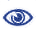# Prague Stringology Conference 2014

Approximation of Greedy Algorithms for Max-ATSP, Maximal Compression, Maximal Cycle Cover, and Shortest Cyclic Cover of Strings

 Abstract: Covering a directed graph by a Hamiltonian path or a set of words by a superstring belong to well studied optimisation problems that prove difficult to approximate. Indeed, the Maximum Asymmetric Travelling Salesman Problem (Max-ATSP), which asks for a Hamiltonian path of maximum weight covering a digraph, and the Shortest Superstring Problem (SSP), which, for a finite language P := {s1, ..., sp}, searches for a string of minimal length having each input word as a substring, are both Max-SNP hard. Finding a short superstring requires to choose a permutation of words and the associated overlaps to minimise the superstring length or to maximise the compression of P. Hence, a strong relation exists between Max-ATSP and SSP since solving Max-ATSP on the Overlap Graph for P gives a shortest superstring. Numerous works have designed algorithms that improve the approximation ratio but are increasingly complex. Often, these rely on solving the pendant problems where the cover is made of cycles instead of single path (Max-CC and SCCS). Finally, the greedy algorithm remains an attractive solution for its simplicity and ease of implementation. Its approximation ratios have been obtained by different approaches. In a seminal but complex proof, Tarhio and Ukkonen showed that it achieves 1/2 compression ratio for Max-CC. Here, using the full power of subset systems, we provide a unified approach for proving simply the approximation ratio of a greedy algorithm for these four problems. Especially, our proof for Maximal Compression shows that the Monge property suffices to derive the 1/2 tight bound.

 Download paper:PDF BibTeX reference
 Download presentation: